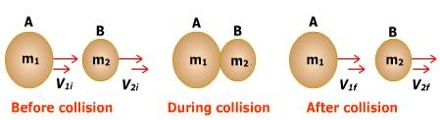# One-dimensional Collision

One-dimensional Collision

When the children play marbles, then if one marble collides with another marble and after the collision if the marbles move in a straight line, then that collision is called one-dimensional collision. That means, if the relative motion of the two colliding bode is along the same straight line below and after the collision, then that collision is called one dimensional collision.

Suppose two particles of masses m1 and m2 are moving in a straight line along x-axis with velocities v01 and v02 respectively (Figure]. Here v01 > v02. At one time the first body hits the second body from behind and afterwards the two particles move along the same straight line with velocities v1 and v2 respectively.Here action force applied on the body of mass m2 is F1 and the mass m2 also creates reaction force F2 on m1.

Again, suppose if the duration of the action and reaction is t,

then sum of initial momentum of the two particles = m1v01 + m2v02 … … … (1)

and the sum of the final momentum of the two particles = m1v1 + m2v2

According to Newton’s third law of motion,

action = reaction

F2 = – F1

During collision the action and reaction forces act for the same duration.

Suppose, the two bodies move along the same straight line, before and after the collision, with velocities v01 and v02 respectively. Due to action and reaction, accelerations of the two bodies become a1 and a2.

then, F1 = – F2

or, m1a1 = – m2a2

or, m1 [(v1 – v01) / t] = – m2 [(v2 – v02) / t]

or, m1v1 – m1v01 = – m2v2 + m2v02

According to the principle of conservation of momentum,

Momentum before collision = Momentum after collision.

This is the equation of one-dimensional collision.